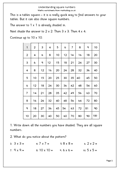# Year 5 maths worksheet: square numbers 1This worksheet has a 10×10 multiplication square which is a brilliant aid to helping with tables, but it is also excellent for showing the pattern of square numbers from 1 to 10.

A square number is made by multiplying a whole number by itself. eg 4 x 4 = 16.

16 is a square number.

It is quite useful to learn the first 10 square numbers off by heart:

1, 4, 9, 16, 25, 36, 49, 64, 81, 100.

We will look more closely at square numbers later.

Square numbers (pg 1)